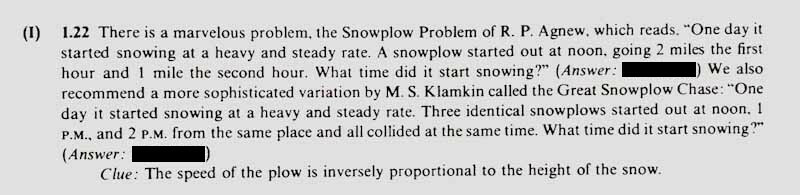In this post, I will walk through a solution to last week’s Riddler from FiveThirtyEight, which concerns a snowplow clearing snow. We are told some details about how the snow falls and how the snowplow clears the snow, and then we are asked (somewhat counterintuitively): when did it start snowing?

Here’s the original prompt, copied directly from FiveThirtyEight:

One morning, it starts snowing. The snow falls at a constant rate, and it continues the rest of the day.

At noon, a snowplow begins to clear the road. The more snow there is on the ground, the slower the plow moves. In fact, the plow’s speed is inversely proportional to the depth of the snow — if you were to double the amount of snow on the ground, the plow would move half as fast.

In its first hour on the road, the plow travels 2 miles. In the second hour, the plow travels only 1 mile.

When did it start snowing?

As the original poster on FiveThirtyEight notes, this problem has been around for a long time. The first time I saw this problem was when I was taking a physics math methods course at Wash U. This problem was presented along with its somewhat more difficult big brother. Here’s both versions, which can be found in Bender and Orszag’s Advanced Mathematical Methods for Scientists and Engineers:The text provides the solutions, which I have blacked out here. Click on the image to see the original without hidden answers.

As a final note, this isn’t the first time FiveThirtyEight has posted problems which I saw in that book. The Riddler from August 19th 2016 (about outrunning a goat) happens to be on the same page as the snowplow problem (see problem 1.30 here)!

## Solving the Snowplow Problem (of R.P. Agnew)

Let $$x(t)$$ denote the position of the snowplow at time $$t$$, and let $$h(t)$$ be the height of the snow at time $$t$$. We are told that the speed of the snowplow is inversely proportional to the snow height, so we have that $$dx/dt = k_1/h$$. We are also told that the snow falls at a constant rate, so letting $$t_0$$ be the unknown time that the snowfall started (for which we would like to solve), we have that $$h(t) = k_2 (t - t_0)$$. Let $$t_1 = 12~p.m$$ denote the time that the snowplow left. Then, letting $$k = k_1/k_2$$, we have that:

$\frac{dx}{dt} = \frac{k}{t - t_0} \qquad \text{for t\geq t_1}.$

Solving the differential equation, we have:

$x(t) = k \int_{t_1}^{t}\frac{dt}{t-t_0} = k \ln\left(\frac{t - t_0}{t_1 - t_0}\right).$

We are told that the plow travels $$2$$ miles in the first hour and $$1$$ mile in the second hour. So, $$x(t_1 + 1) = 2$$ and $$x(t_1 + 2) = 2 + 1 = 3$$. Hence,

$2 = k \ln\left(\frac{(t_1 + 1) - t_0}{t_1 - t_0}\right)$

and

$3 = k \ln\left(\frac{(t_1 + 2) - t_0}{t_1 - t_0}\right).$

Combining these two expressions and rearranging, we obtain:

$3 \cdot \ln\left(\frac{(t_1 + 1) - t_0}{t_1 - t_0}\right) = 2 \cdot \ln\left(\frac{(t_1 + 2) - t_0}{t_1 - t_0}\right).$

from which it follows that:

$((t_1 + 1) - t_0)^3 \cdot (t_1 - t_0)^2 = ((t_1 + 2) - t_0)^2 \cdot (t_1 - t_0)^3.$

Now, recall that we are trying to solve for $$t_0$$, and that we must have $$t_0 < t_1$$ (since the snow started falling before the snowplow left). So we can safely reduce this to:

$((t_1 + 1) - t_0)^3 = ((t_1 + 2) - t_0)^2 \cdot (t_1 - t_0).$

Using that $$t_1 = 12$$ we must solve:

$(13 - t_0)^3 = (14 - t_0)^2 \cdot (12 - t_0),$

which is equivalent to:

$0 = t^2 - 25t + 155,$

which has solutions $$t_0 = \frac{25}{2} \pm \frac{\sqrt{5}}{2}.$$ Since we must have $$t_0 < 12$$, it follows that

$t_0 = \frac{25}{2} - \frac{\sqrt{5}}{2} \approx 11.382$

Finally, we convert this into a human readable form, and find that it started snowing at about 11:23 a.m.